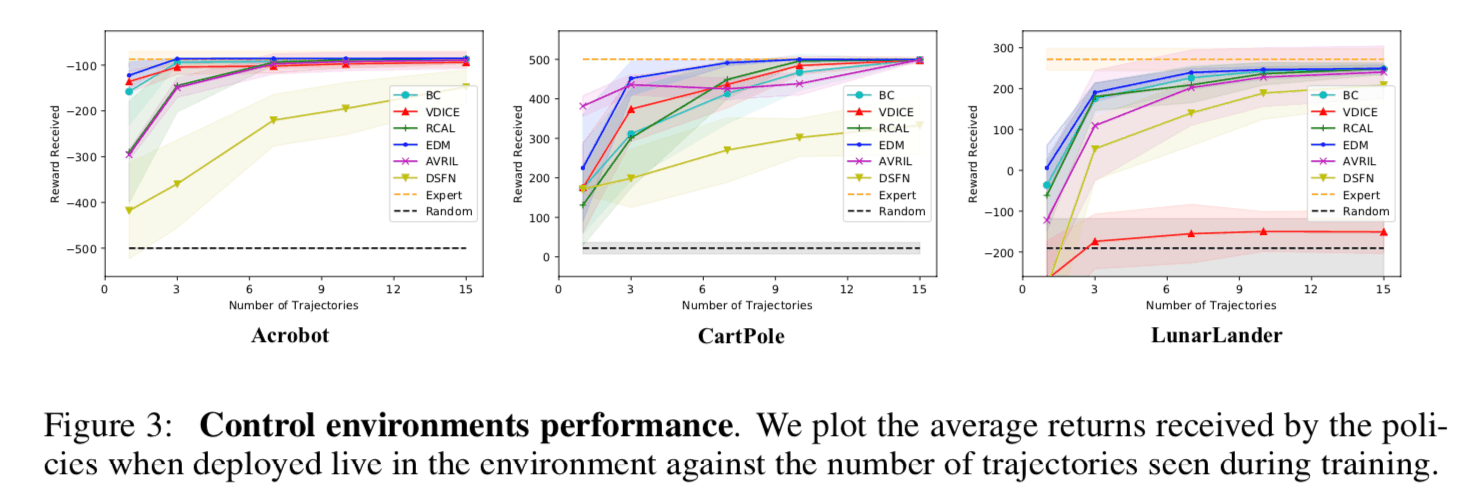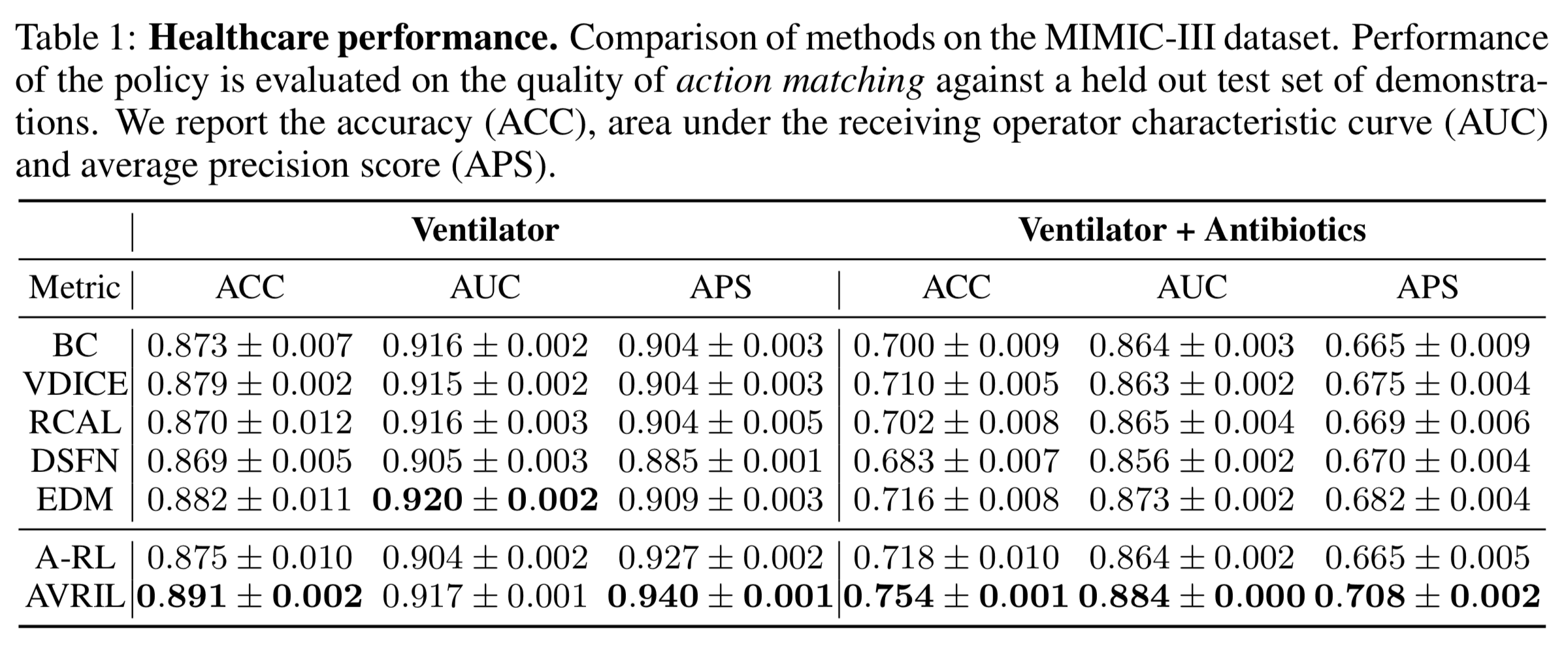# Catch up on the latest AI articles# Scalable Bayesian Inverse Reinforcement Learning! A First Step Toward Realizing Secure Imitation Learning!

3 main points
✔️ Research on Bayesian inverse reinforcement learning that can quantify the uncertainty in estimating the reward function
✔️ Proposed a learning algorithm that can be applied to large-scale state-space problems by avoiding MCMC iterations, which is a bottleneck of conventional Bayesian inverse reinforcement learning
✔️ Achieves higher inference performance than benchmark methods in comparative experiments on medical diagnostic datasets

The images used in this article are from the paper, the introductory slides, or were created based on them.

## first of all

Inverse reinforcement learning is a method for learning a reward function from behavioral data of a desired task. Furthermore, by performing reinforcement learning using the learned reward function, it is possible to learn strategies to imitate the behavioral data. This framework is called Imitation learning and is known as a method for learning measures that represent human decision making while automating the design of the reward function, which has been a problem in conventional reinforcement learning.

This research is a study on Bayesian inverse reinforcement learning, which enables imitation learning considering the uncertainty of the estimation accuracy of the reward function by obtaining the posterior distribution of the reward function. The advantage of estimating the uncertainty of the estimation accuracy of the reward function is to assess the safety of the learned measures and to plan future data collection. Traditional Bayesian inverse reinforcement learning (Ramachandran & Amir, 2007) uses an algorithm based on the Markov chain Monte Carlo method (MCMC ) to sample multiple times from the posterior distribution and estimate the The sample mean of the reward function can be estimated as the sample mean of the posterior distribution. However, in the process of computing the sample mean of the reward functionQ-values(i.e., the sample mean of the reward function) must be iteratively computed in the process of computing the sample mean of the reward function.

In this study, we represent the posterior distribution of the reward function and the Q-function calculated from the expected value of the reward function by different function approximators, and optimize the objective function derived fromVariational Inference The proposed algorithm updates the parameters of the two function approximators simultaneously by optimizing the objective function derived from the Q-function over the constraints expressing the relationship between the reward function and the Q-function. Since the proposed method directly models the Q-function with respect to the expected value of the reward function, it has an advantage that the strategy can be computed without performing a computationally expensive MCMC. Furthermore, the proposed method can be applied to problems in continuous state space and continuous action space because the Q-function calculated from the posterior distribution of the reward function and the expected value of the reward function is learned as a function approximator.

In this study, the superiority of the proposed method is demonstrated based on the experimental results of three tasks: a Grid World task, a control task on a continuous state space, and an off-line medical diagnosis task. offline medical diagnosis task. In particular, it is shown that the proposed method can achieve better inference performance than existing benchmark methods for offline inverse reinforcement learning in the offlinemedical diagnosis task.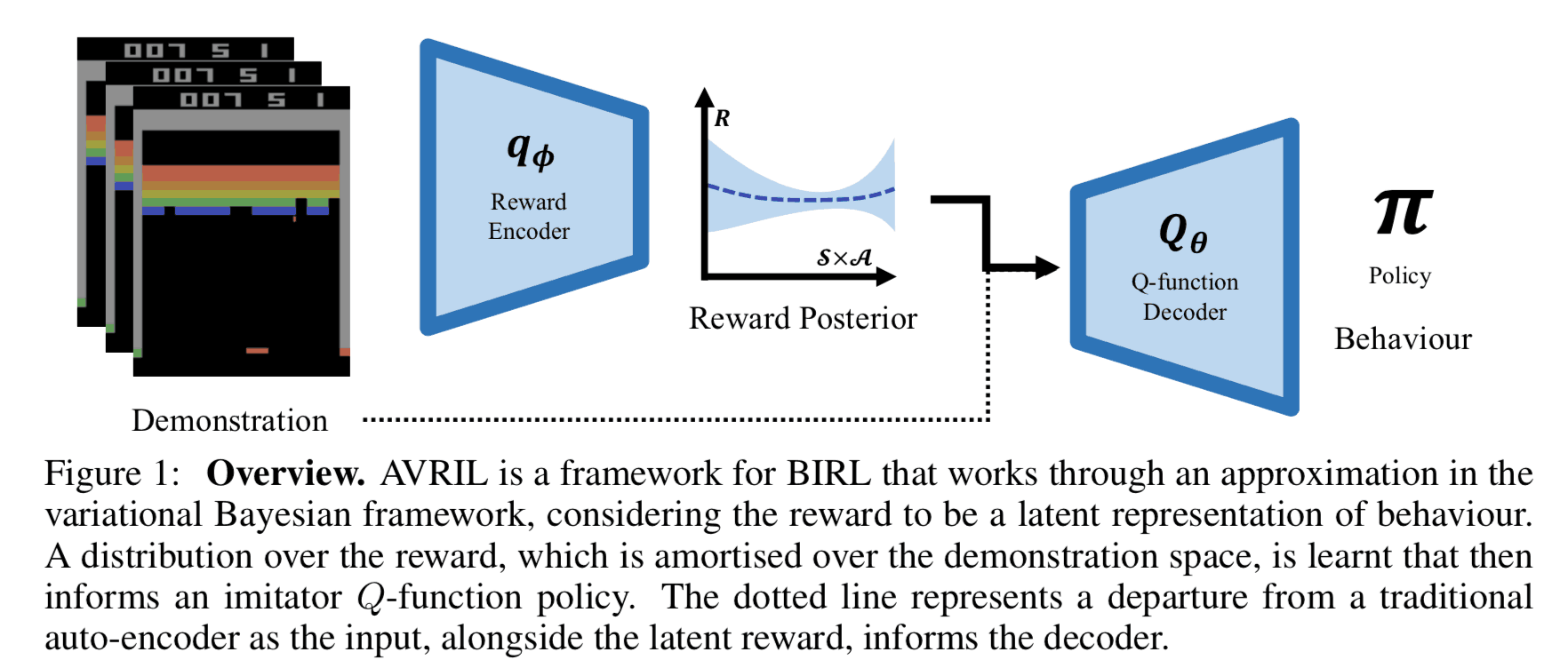## previous work

In this section, we outline prior work on Bayesian inverse reinforcement learning (Ramachandran & Amir, 2007). In the following, we assume that a set of behavioral data $\mathcal{D} = \{\tau_1, \tau_2, \cdots, \tau_N \}$ for the desired task has been obtained. Each behavioral data $\tau_n$ is represented as a series of data consisting of pairs of states $s\in\mathcal{S}$ and behaviors $a\in\mathcal{A}$.

$$\tau = \{(s_0, a_0), (s_1, a_1), \cdots, (s_T, a_T)\} \tag{a}$$

In Bayesian inverse reinforcement learning, the state action value in state $S$. Q^{\pi}_{R}$is maximized in state$s$, and the optimal strategy$\pi(s)$is the optimal strategy$\pi(s)$is to select the action$a$that maximizes the state action value is the optimal policy$\pi(s)$. $$\pi(s)\in \underset{a\in\mathcal{A}}{\operatorname{argmax}}}Q^{\pi}_{R}(s, a) \tag{b}$$ where$Q^{\pi}_{R}$is the Q function ( state-action value function), and Perform action$a$in state$s$in an environment with a known reward function$R$, and then follows the policy$\pi$. in the case where The expected total reward to be earned. The total expected reward for the action$R$is $$Q^{\pi}_{R}(s, a) = \mathbb{E}_{\pi, \mathcal{T}} \left\lbrack \sum_{t}\gamma^{t}R(s_t, a_t)\middle| s_0=s, a_0=a, \pi \right\rbrack \tag{c}$$$

where $\gamma \in\lbrack 0, 1)$ is the discount rate, a hyperparameter that expresses the extent to which future earned compensation is considered in current decisions.

In Bayesian inverse reinforcement learning, the posterior distribution of the reward function $P(R\mid\mathcal{D})$ is estimated under the action data $\mathcal{D}$. The posterior distribution can be calculated by Bayes' theorem as follows.

$$P(R\mid \mathcal{D}) = \frac{P(\mathcal{D}\mid R)P(R)}{P(\mathcal{D})} \tag{d}$$

The $P(R)$ is the prior distribution of the reward function, and represents prior knowledge about the reward function by making appropriate choices. Also, $P(\mathcal{D}\mid R)$ is the likelihood function, a quantity that represents the plausibility of obtaining the observed values of the arguments from the assumed model. In Bayesian inverse reinforcement learning, it is assumed that the likelihood function can be represented by a Boltzmann distribution with the Q function as the energy function.

\begin{align}P(\mathcal{D}\mid R) &= \prod_{n=1}^N P(\tau_n \mid R) \cp&\propto \prod_{n=1}^N \sum_{(s, a)\in\tau_n}\exp\left(\beta Q^{\pI}_{R}(s, a)\tag{e}} \end{align} \tag{e} where\beta\in\lbrack 0, 1)$is the inverse temperature, a hyperparameter that represents how well the demonstrator chooses the optimal behavior. In Bayesian inverse reinforcement learning, the expected value of the reward function is approximated by the sample mean from the posterior distribution, and the Q function is estimated using the reward function obtained as the sample mean. In this case, sampling from the posterior distribution is performed using MCMC, which repeatedly selects samples using a rejection condition based on the ratio of the posterior distribution of the reward function. On the other hand, as we saw in equation (e), the likelihood function depends on the Q-function in Bayesian inverse reinforcement learning, so the Q-function needs to be calculated every time the rejection condition is computed. Therefore, it is difficult to apply this method to the problem setting that deals with a large-scale state space from the viewpoint of computational complexity. ## proposed method In this paper, we propose an algorithm that does not require sampling from the posterior distribution of the reward function, which is a bottleneck of conventional Bayesian inverse reinforcement learning. In this section, we explain the derivation process of the objective function of the proposed method. First, the problem of finding the approximate posterior distribution$q_{\mid\mathcal{D})$of the posterior distribution$P(R\mid\mathcal{D})$can be written as a problem of minimizing the KL divergence between the distributions where$q_{{C}}$is the KL divergence between the distributions.(6) Equation can be expanded according to the definition of KL divergence and transformed into the following equation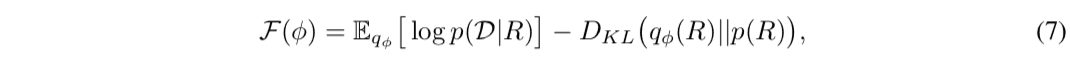where$\mathcal{F}(\phi)$is a quantity calledVariational Lower BOund (Evidence Lower BOund, ELBO) and it is known that the maximization problem of the variational lower bound is equivalent to the optimization problem in equation (6). Furthermore, by replacing the likelihood function in the expectation of the first term in Eq. (7) by Eq. (e), the objective function can be written down as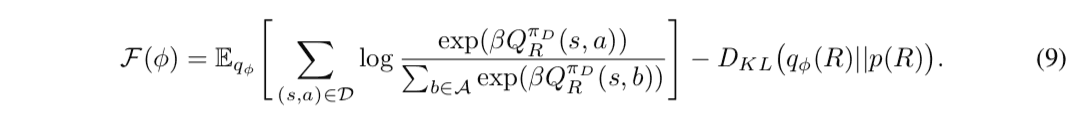Here, since it is difficult to calculate the expectation value calculation for the approximate posterior distribution$q_{\phi}$of equation (9) analytically, it is calculated approximately by the sample mean from$q_{\phi}$. At this time, since the likelihood function inside the expected value depends on the Q function, it is necessary to calculate the Q function for each sample of the reward function to calculate the sample average. Therefore, the iterative computation of the Q-function becomes a bottleneck, and there remains a problem from the viewpoint of computational complexity in the problem setting where a large number of states are handled. Therefore, in the proposed method, we introduce a function approximator$Q_{\mathbb{E}_{R\sim q_{\phi}}\lbrack R \rbrack$, which represents the Q function for the expected value of the reward function$\mathbb{E}_{R\sim q_{\phi}}\lbrack$with respect to the approximate posterior distribution$q_{\phi}$. }}$ is trained at the same time. In this case, the update of the Q function and the reward functionBellman equation The Bellman equation is a recursive equation of the Q-function. The Bellman equation is an expression that gives a recursive definition of the Q function, and can be written down using the reward function $R$ as

$$R(s, a) = \mathbb{E}_{\pi, \mathcal{T}}\lbrack Q(s, a) - \gamma Q(s^{\prime}, a^{\prime}) \rbrack \tag{f}$$

Therefore, we may add as a constraint that the negative log-likelihood of $q_{\phi}$ is less than a sufficiently small positive number $\epsilon$ in the value of the reward function calculated using the function approximator $Q_{\theta}$ that represents the Q function.

$$- \log q_{\phi}\left( \mathbb{E}_{\pi, \mathcal{T}}\lbrack Q(s, a) - \gamma Q(s^{\prime}, a^{\prime}) \rbrack\right) < \epsilon \tag{g}$$

Based on the above, we can obtain the optimization problem of equation (10) as an approximation of the optimization problem of equation (9). (The following equation (10) is quoted as it is from the paper, but it seems that there is a typographical error in the notation of the constraint condition. The constraint condition is supposed to be correctly expressed by equation (g)).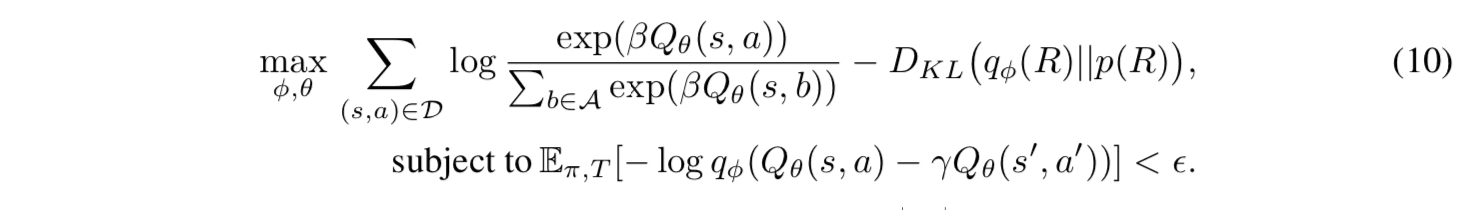Furthermore, by rewriting the objective function in equation (10) using the Lagrange undetermined multiplier method and approximating the expectation of the constraints by the sample mean of the behavioral data, we obtain the objective function $\mathcal{F}(\phi, \theta, \mathcal{D})$.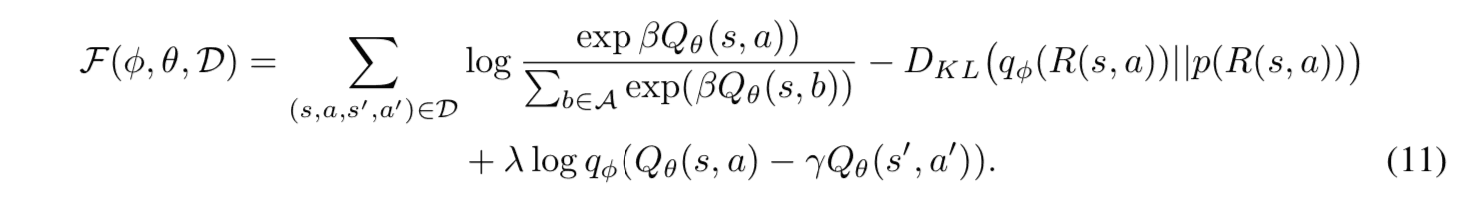where $\lambda$ is a positive constant that determines the strength of the influence of the constraints. The proposed method iteratively updates the model based on the gradients with respect to $\theta$ and $\phi$ in equation (10). The pseudo code of the learning algorithm is shown below.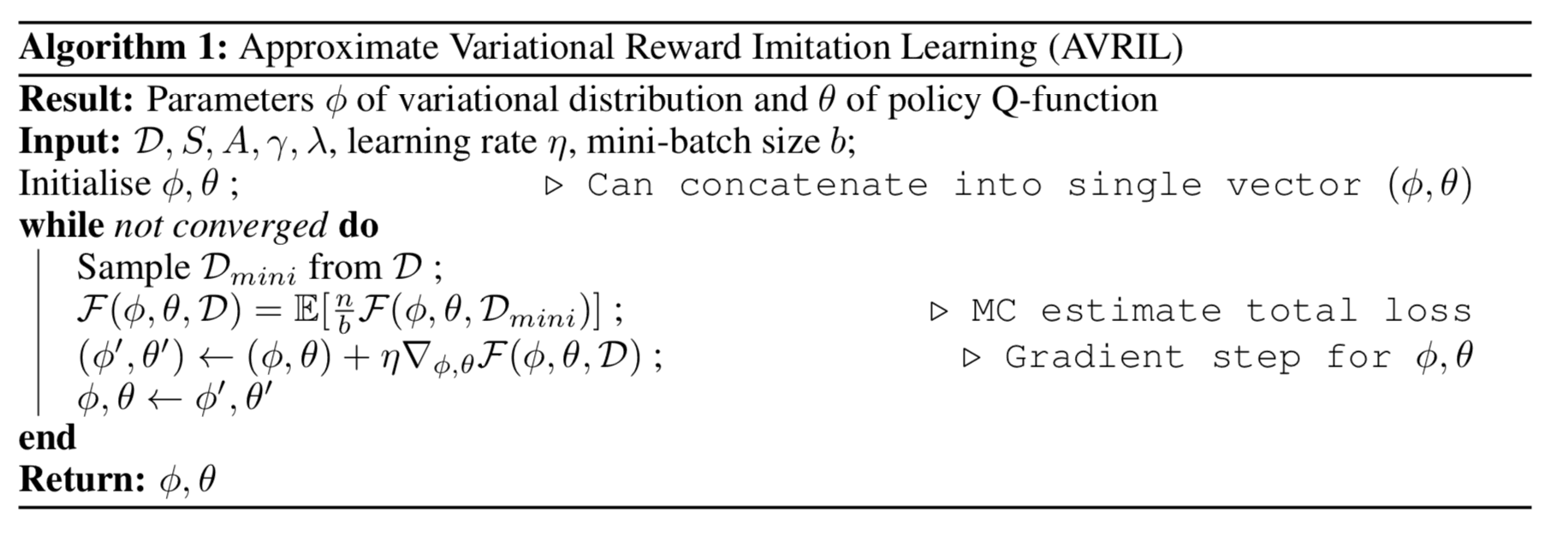## experiment

In this study, we propose a Grid World task, a control task on a continuous state space, an online medical diagnosis task. the superiority of the proposed method is shown The following section describes the contents and results of each experiment. This section describes the contents and results of each experiment.

### Grid World

The goal of this task is to reach the target point by transitioning between states arranged on a board. In the figure below, a) is the true reward function designed manually, b) is the frequency distribution of the teacher data visiting each state, c) is the sample mean of the learned reward function, and d) is the standard deviation of the samples visualized in a heatmap. It can be said that a) and c) are close to the true reward function. Comparing b) and d), we can see that the standard deviation of samples from the posterior distribution is larger in the region with low visit frequency in the teacher data (the upper right region in the figure), indicating that the estimation uncertainty of the reward function is high. Thus, Bayesian inverse reinforcement learning has the advantage that the "reliability" of the estimation of the reward function can be quantified, and the safety of the strategy can be evaluated.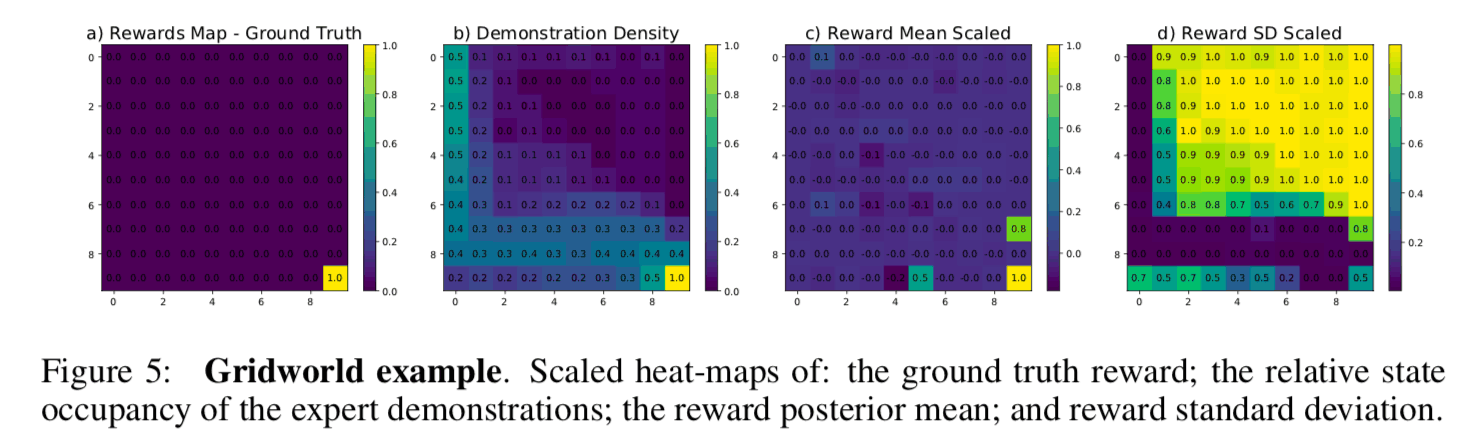### Control Tasks on Continuous State Space

In this experiment, three different robot control tasks (Acrobat, CartPole, and LunarLander) on OpenAI gym are compared with the benchmark methodSample complexity with the benchmark methods on three different robot control tasks (Acrobat, CartPole, and LunarLander). The sample complexity is roughly defined as the amount of teacher data required for the model to reach a sufficient inference performance, and the lower the sample complexity, the less teacher data is required to train the model. The figure below shows a plot of the number of teacher data on the horizontal axis and the total reward on the vertical axis for each control task. From this figure, we can see that the proposed method (AVRIL, Pink) achieves the same performance as the experts in all tasks with the same number of teacher data as the other benchmark methods. Since the other benchmark methods are based on point estimation of the reward function, it can be said that the proposed method can learn more informative results (posterior distribution of the reward function) with the same amount of teacher data.